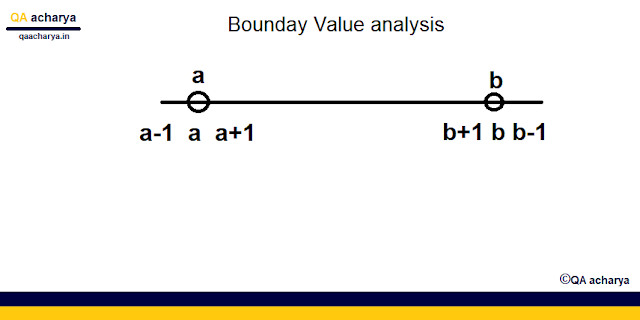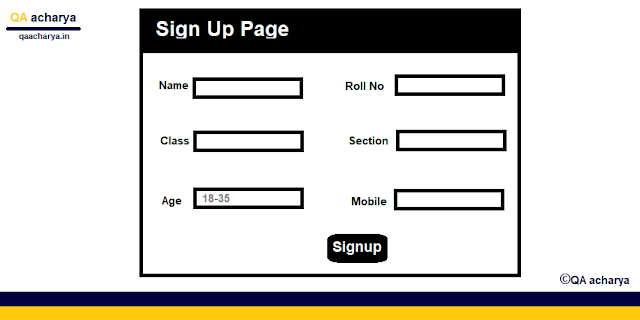# Boundary Value Analysis in Software Testing with Example.
Boundary Value Analysis in Software Testing (BVA)

Boundary value analysis is a (software testing) black box Testing technique or specification-based testing technique. This technique is used to test the boundary values. It is widely recognized that input values at the extreme ends of the input domain cause more chances of errors in the application.
While testing the boundary value analysis the main aim of the software tester is to check whether the application is providing the correct input or not when entering the boundary values.
• Boundary value analysis was done after the compilation of Equivalence Partitioning.
• Equivalence class Partitioning plays an important role in boundary testing.Fig. Boundary value analysis example

What is boundary value?
A boundary value is one that contains the limits of a variable (Upper and lower ). For Example, there is a student registration page and an age field is there which accept student aged 18 as the minimum and 35 as the maximum. Here (18 -35) are the boundary value for the field.

How to do Boundary Value Testing?
If the field to be tested accept the range of value from a to b then test the field by (a-1, a a+1 and b-1, b,b+1)Fig.  Boundary value testing

Example of Boundary Value Analysis
In the below student sign-up page the boundary value for the age field is 18 and 35 let's try to understand the real analysis by this example.Fig. Boundary value analysis Example

Boundary Value : 18(Minimum) and 35 (maximum)
using (a-1, a,a+1 and b-1, b,b+1) formula test engineers have to find the range and on the basis of this, they have to write the test cases.
(17, 18, 19 and 34,35,36).

While writing the test cases for boundary value tester has to identify the range boundary value and then start writing test cases.

Example - Test Cases

• Check the age field by entering the value which is not in the range (ie.17and 36)
• Check the age field by entering the exact boundary value (ie . 18and 35 )
• Check the age field by entering the value which is within the boundary value (17 to 34)
The various boundary value analysis advantage is given below
• The boundary value analysis (BVA) technique of testing is very easy to use and remember.
• The overall time taken to execute the test cases is reduced.
• Generation of test cases through BVA is minimal
A list of the disadvantage of boundary value analysis is given below.
• BVA does not test all possible inputs.
• BVA does not test the combination of inputs.

Hope !! The above tutorial on boundary value analysis is helpful for You ...
Team
QA acharya

Share Your Knowledge With Us -